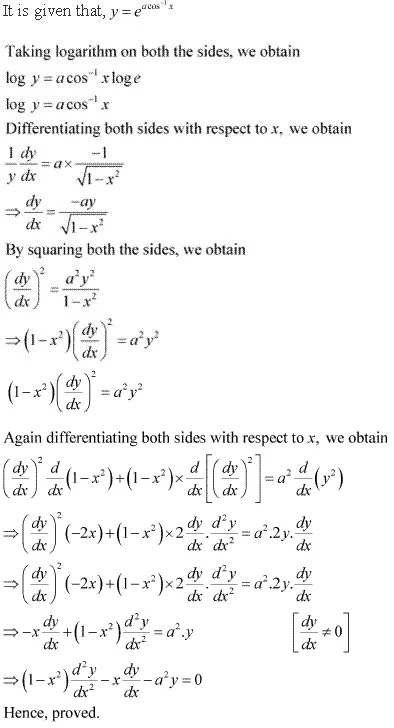# Class 12 Maths NCERT Solutions for Chapter 5 Continuity and Differentiability Miscellaneous Exercise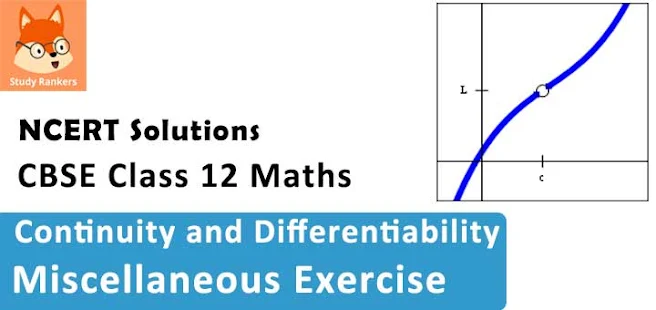### Continuity and Differentiability Miscellaneous Exercise Solutions

1. Differentiate w.r.t. x the function (3x2 – 9x + 5)9

Solution

Let y =  (3x2 – 9x + 5)9
Using chain rule, we obtain
dy/dx = d/dx  (3x2 - 9x + 5)9
= 9(3x2 - 9x + 5)9 . d/dx (3x2 - 9x + 5)
= 9(3x2 - 9x + 5)8 . (6x - 9)
= 9(3x2 - 9x + 5)8 . 3(2x - 3)
= 27(3x2 - 9x + 5)8 (2x - 3)

2. Differentiate w.r.t. x the function sin3 x + cos6 x

Solution

Let y = sin3 x + cos6 x
∴ dy/dx = d/dx (sin3 x) + d/dx (cos6 x)
= 3 sin2 x . d/dx (sin x) + 6 cos5 x . d/dx  (cos x)
= 3 sin2 x . cos x + 6 cos5 x . (-sin x)
= 3 sin x cosx(sin x - 2 cos4 x)

3. Differentiate w.r.t. x the function (5x)3cos 2x

Solution

Let y =  (5x)3cos 2x
Taking logarithm on both the sides, we obtain
log y = 3 cos 2x log 5x
Differentiating both sides with respect to x, we obtain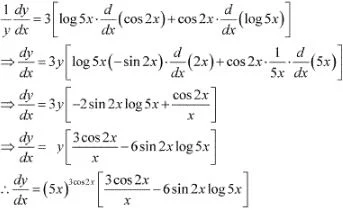4. Differentiate w.r.t. x the function sin-1 (x√x), 0 ≤ x ≤ 1

Solution

Let y = sin-1 (x√x)
Using chain rule, we obtain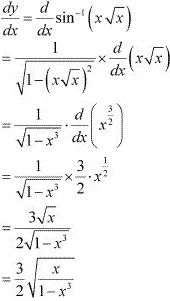5. Differentiate w.r.t.  the function, -2 < x < 2

Solution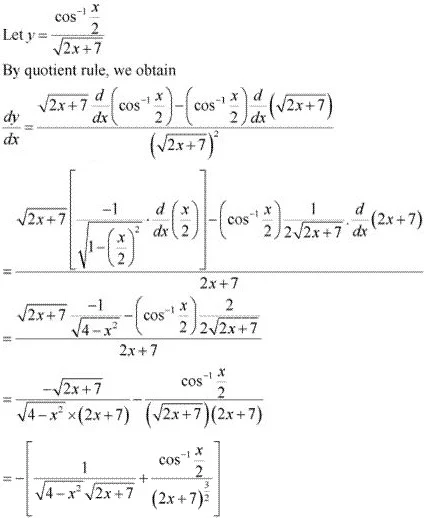6. Differentiate w.r.t. x the function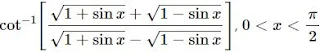Solution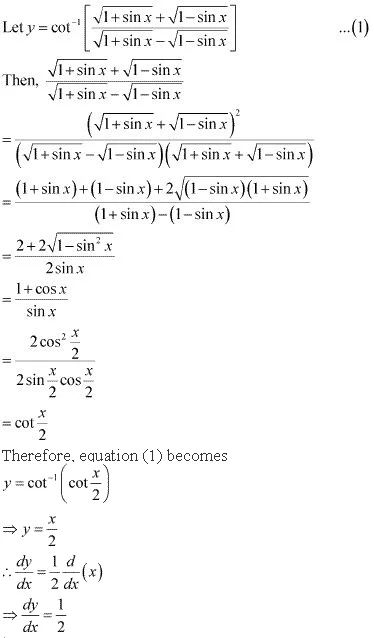7. Differentiate w.r.t. x the function (log x)log x  , x > 1
Solution
Let y = (log x)log x
Taking logarithm on both the sides, we obtain
log  y = log x . log(log x)
Differentiating both sides with respect to x, we obtain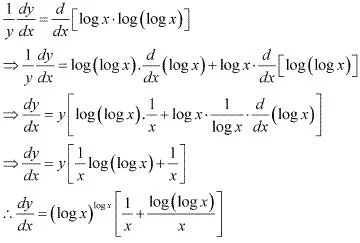8. Differentiate w.r.t. x the function cos (a cos x + b sin x), for some constant a and b.
Solution
Let y = cos(a cos x + b sin x)
By using chain rule, we obtain
dy/dx = d/dx  cos(a cos x + b sin x)
⇒ dy/dx = - sin(a cos x + b sin x). d/dx (a cos x + b sin x)
= - sin (a cos x + b sin x) . [a(-sin x) + b cos x]
= (a sin x - b cos x) . sin (a cos x + bsin x)

9. Differentiate w.r.t. x the function (sin x - cos x)(sin x - cos x)  , π/4 < x < 3π/4
Solution
Let y = (sin x - cos x)(sin x - cos x)
Taking logarithm on both the sides, we obtain
log y = log[(sin x - cos x)(sin x - cos x)  ]
⇒ log y = (sin x - cos x) . log(sin x - cos x)
Differentiating both sides with respect to x, we obtain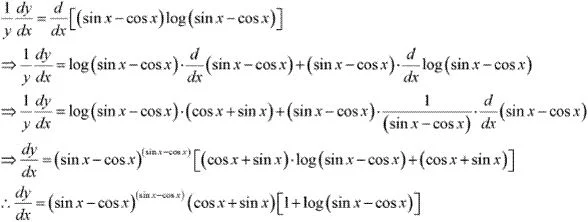where sin x > cos x

10. Differentiate w.r.t. x the function xx + xa + ax + aa, for some fixed a > 0 and x > 0
Solution
Let y = xx + xa + ax + aa
Also, let xx = u, xa = v, ax = w, and aa = s
∴ y = u + v + w + s
⇒ dy/dx = du/dx + dv/dx + dw/dx + ds/dx ...(1)
u = xx
⇒ log u = log xx
⇒ log u = x log x
Differentiating both sides with respect to x, we obtain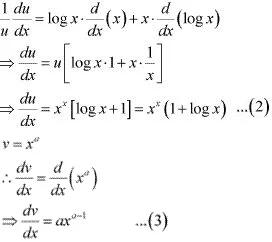w = ax
⇒ log w = log ax
⇒ log w = x log a
Differentiating both sides with respect to x, we obtain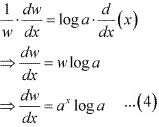s = aa
Since a is constant, aa is also a constant.
ds/dx = 0 ...(5)
From (1), (2), (3), (4) and (5), we obtain
dy/dx = xx (1 + log x) + axa-1  + ax log a + 0
= xx (1 + log x) + axa-1 + ax log a

11. Differentiate w.r.t. x the function xx2-3 + (x - 3)x2, for x > 3
Solution
Let y = xx2-3  + (x - 3)x2
Also, let u = xx2-3 and v = (x - 3)x2
∴ y = u + v
Differentiating both sides with respect to x, we obtain
dy/dx = du/dx + dv/dx ...(1)
u = xx2-3
∴ log u = log(xx2-3)
log u = (x2 - 3) log x
Differentiating with respect to x, we obtain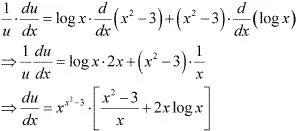Also,
v =(x - 3)x2
∴ log v = log (x - 3)x2
⇒ log v = x2 log (x - 3)
Differentiating both sides with respect to x, we obtain12. Find dy/dx , if y = 12(1 - cos t), x = 10(t - sin t), - π/2 < t < π/2
Solution
It is given that, y = 12 (1 - cost), x = 10(t - sin t)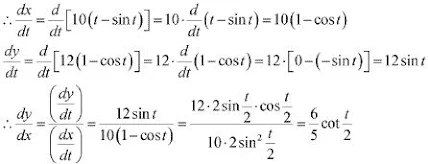13. Find dy/dx , if y = sin-1 x + sin-1 √(1 - x2 ), 0 < x < 1
Solution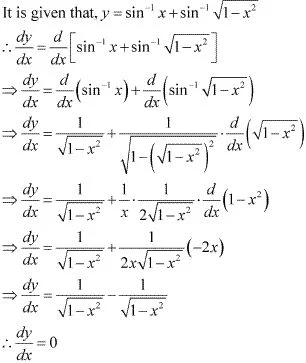14. If x√(1 + y) + y√(1 + x) = 0, for, -1<x<1, prove that dy/dx = 1/(1 + x)2
Solution
It is given that,
x√(1 + y) + y√(1 + x) = 0
⇒ x√(1 + y)  = - y√(1 + x)
Squaring both sides, we obtain
x2 (1 + y) = y2 (1 + x)
⇒ x2 + x2 y = y2 + xy2
⇒ x2 - y2 = xy2 - x2 y
⇒ x2 - y2 = xy(y - x)
⇒ (x + y)(x - y) = xy(y - x)
∴ x + y = -xy
⇒ (1 + x)y = -x
⇒ y = -x/(1 + x)
Differentiating both sides with respect to x, we obtain
y = -x/(1 + x)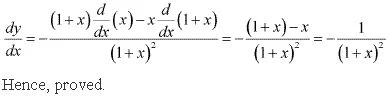15. If (x – a)2 + (y – b)2 = c2, for some c > 0, prove that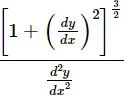is a constant independent of a and b.
Solution
Differentiating both sides with respect to x, we obtain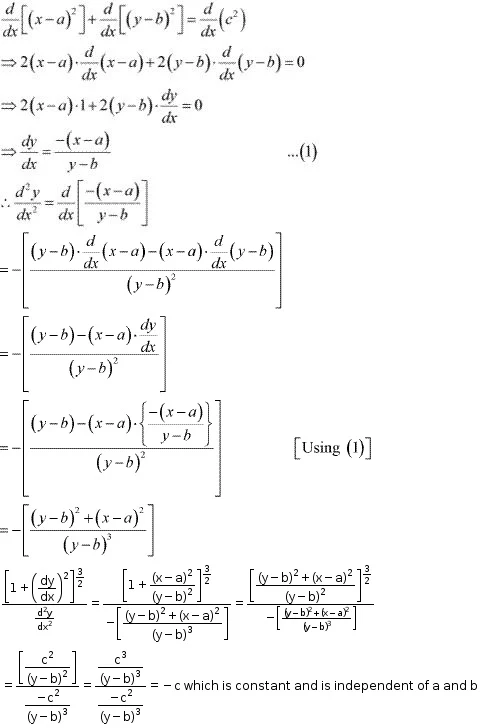Hence, proved.

16. If cos y = x cos (a + y), with cos a ≠ ± 1, prove that dy/dx = [cos2 (a + y)]/ sin a
Solution
It is given that, cos y = x cos(a + y)
∴ d/dx [ cos y] = d/dx [x cos(a + y)]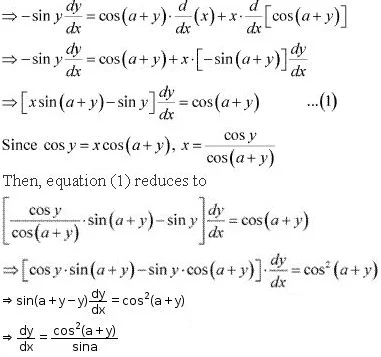17. If x = a (cos t + t sin t) and y = a (sin t – t cos t), find d2 y/dx2  .
Solution
It is given that, x = a(cost + t sin t) and y = a(sin t - t cos t)
∴ dx/dt = a . d/dt (cos t + t sin t)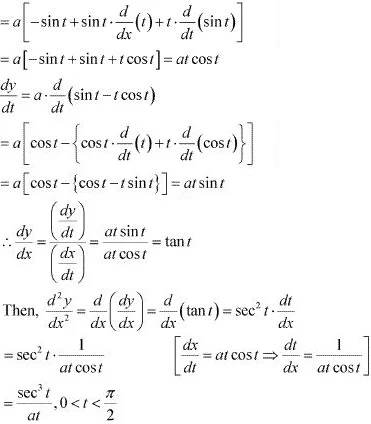18. If f (x) = |x|3, show that f ″(x) exists for all real x and find it.
Solution
It is known that, |x| =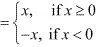Therefore, when x ≥ 0, f(x) = |x|3 = x3
In this case, f '(x) = 3x2 and hence, f ''(x) = 6x
When x < 0, f(x) = |x|3 = (-x)3 = -x3
In this case, f '(x) = -3x2 and hence, f ''(x) = -6x
Thus, for f(x) = |x|3 , f ''(x) exists for all real x and is given by,
f ''(x)19. Using mathematical induction prove that d/dx (xn ) = nxn-1 for all positive integers n.
Solution
To prove : p(n) : d/dx (xn ) = nxn-1 for all positive integers n
for n = 1,
p(1) : d/dx (x) = 1 = 1 .x1-1
P(n) is true for n = 1
Let P(k) is true for some positive integer k.
That is, P(k) : d/dx (x4 ) = kxk-1
It has to be proved that P(k + 1) is also true.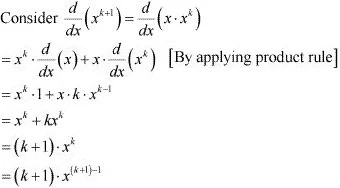Thus, P(k + 1) is true whenever P (k) is true.
Therefore, by the principle of mathematical induction, the statement P (n) is true for every positive integer n.
Hence, proved.

20. Using the fact that sin (A + B) = sin A cos B + cos A sin B and the differentiation, obtain the sum formula for cosines
Solution
sin (A + B) = sin A cos B + cos A sin B
Differentiating both sides with respect to x, we obtain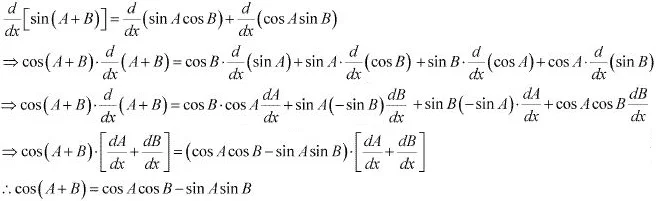21. Does there exist a function which is continuous everywhere but not differentiable at exactly two points? Justify your answer ?
Solution
Yes,
Consider the function f(x) = |x - 1| + |x - 2|
Since we know that the modulus function is continuous everywhere, so there sum is also continuous.
Therefore, function f is continuous everywhere
Now, let us check the differentiability of f(x) at x = 1, 2
At x = 1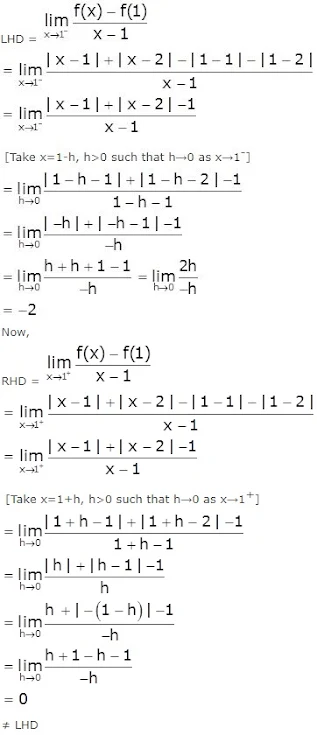Therefore, f is not differentiable at x = 1.
At x = 2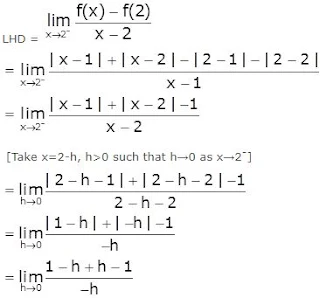= -0
Now,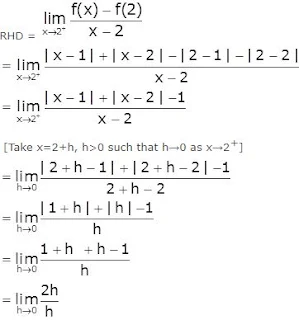= 2
≠ LHD
Therefore, f is not differentiable at x = 2.
Hence, f is not differentiable at exactly two points.

22. If y =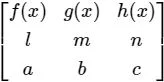, prove that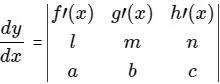Solution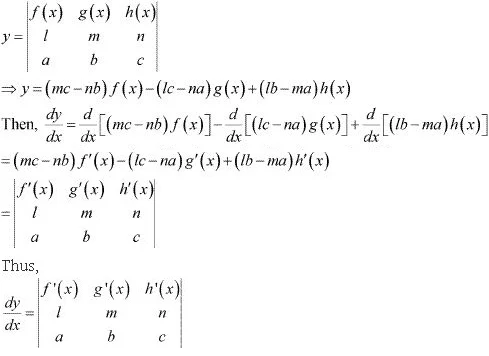23. If y = ea cos-1  x   , -1 < = x < 1 show that (1 - x2 ) d2 y/dx2  - x . dy/dx  - a2 y = 0
Solution